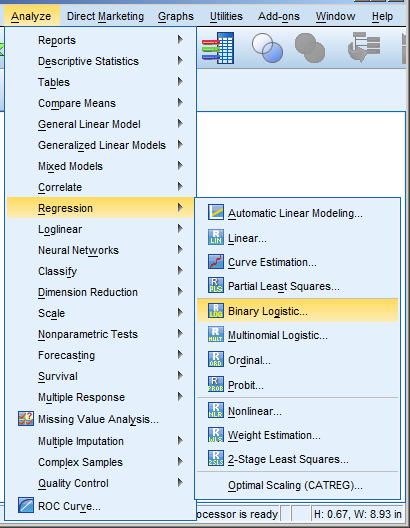# logistic regression

### Effect Size Statistics in Logistic Regression

May 18th, 2015 by

Effect size statistics are expected by many journal editors these days.

If you’re running an ANOVA, t-test, or linear regression model, it’s pretty straightforward which ones to report.

Things get trickier, though, once you venture into other types of models.` (more…)`

### Generalized Linear Models in R, Part 3: Plotting Predicted Probabilities

July 2nd, 2014 by

by David Lillis, Ph.D.

In our last article, we learned about model fit in Generalized Linear Models on binary data using the glm() command. We continue with the same glm on the mtcars data set (regressing the vs variable on the weight and engine displacement).

Now we want to plot our model, along with the observed data.

Although we ran a model with multiple predictors, it can help interpretation to plot the predicted probability that vs=1 against each predictor separately.  So first we fit a glm for only (more…)

### Generalized Linear Models in R, Part 2: Understanding Model Fit in Logistic Regression Output

June 24th, 2014 by

by David Lillis, Ph.D.

In the last article, we saw how to create a simple Generalized Linear Model on binary data using the glm() command. We continue with the same glm on the mtcars data set (more…)

### Generalized Linear Models in R, Part 1: Calculating Predicted Probability in Binary Logistic Regression

June 18th, 2014 by

by David Lillis, Ph.D.

Ordinary Least Squares regression provides linear models of continuous variables. However, much data of interest to statisticians and researchers are not continuous and so other methods must be used to create useful predictive models.

The glm() command is designed to perform generalized linear models (regressions) on binary outcome data, count data, probability data, proportion data and many other data types.

In this blog post, we explore the use of R’s glm() command on one such data type. Let’s take a look at a simple example where we model binary data.

### SPSS Procedures for Logistic Regression

May 15th, 2014 by

Need to run a logistic regression in SPSS? Turns out, SPSS has a number of procedures for running different types of logistic regression.

Some types of logistic regression can be run in more than one procedure.  For some unknown reason, some procedures produce output others don’t.  So it’s helpful to be able to use more than one.

### Logistic RegressionLogistic Regression can be used only for binary dependent (more…)

### Member Training: Discrete Time Event History Analysis

February 1st, 2014 by

What is the relationship between predictors and whether and when an event will occur?This is what event history (a.k.a., survival) analysis tests.

There are many flavors of Event History Analysis, though, depending on how time is measured, whether events can repeat, etc.

In this webinar, we discussed many of the issues involved in measuring time, including censoring, and introduce one specific type of event history model: the logistic model for discrete time events.

Note: This training is an exclusive benefit to members of the Statistically Speaking Membership Program and part of the Stat’s Amore Trainings Series. Each Stat’s Amore Training is approximately 90 minutes long.